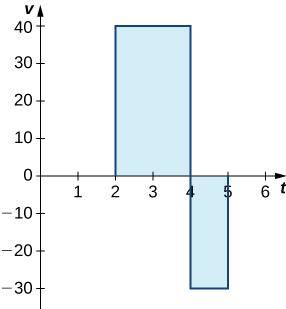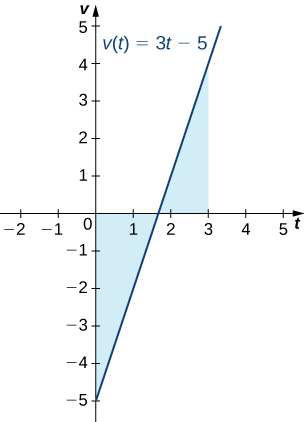# 5.4 Integration formulas and the net change theorem  (Page 2/8)

 Page 2 / 8The graph shows speed versus time for the given motion of a car.

Just as we did before, we can use definite integrals to calculate the net displacement as well as the total distance traveled. The net displacement is given by

$\begin{array}{cc}{\int }_{2}^{5}v\left(t\right)dt\hfill & ={\int }_{2}^{4}40dt+{\int }_{4}^{5}-30dt\hfill \\ & =80-30\hfill \\ & =50.\hfill \end{array}$

Thus, at 5 p.m. the car is 50 mi north of its starting position. The total distance traveled is given by

$\begin{array}{}\\ \\ {\int }_{2}^{5}|v\left(t\right)|dt\hfill & ={\int }_{2}^{4}40dt+{\int }_{4}^{5}30dt\hfill \\ & =80+30\hfill \\ & =110.\hfill \end{array}$

Therefore, between 2 p.m. and 5 p.m., the car traveled a total of 110 mi.

To summarize, net displacement may include both positive and negative values. In other words, the velocity function accounts for both forward distance and backward distance. To find net displacement, integrate the velocity function over the interval. Total distance traveled, on the other hand, is always positive. To find the total distance traveled by an object, regardless of direction, we need to integrate the absolute value of the velocity function.

## Finding net displacement

Given a velocity function $v\left(t\right)=3t-5$ (in meters per second) for a particle in motion from time $t=0$ to time $t=3,$ find the net displacement of the particle.

Applying the net change theorem, we have

$\begin{array}{ll}{\int }_{0}^{3}\left(3t-5\right)dt\hfill & =\frac{3{t}^{2}}{2}-5t{|}_{0}^{3}\hfill \\ \\ & =\left[\frac{3{\left(3\right)}^{2}}{2}-5\left(3\right)\right]-0\hfill \\ & =\frac{27}{2}-15\hfill \\ & =\frac{27}{2}-\frac{30}{2}\hfill \\ & =-\frac{3}{2}.\hfill \end{array}$

The net displacement is $-\frac{3}{2}$ m ( [link] ).The graph shows velocity versus time for a particle moving with a linear velocity function.

## Finding the total distance traveled

Use [link] to find the total distance traveled by a particle according to the velocity function $v\left(t\right)=3t-5$ m/sec over a time interval $\left[0,3\right].$

The total distance traveled includes both the positive and the negative values. Therefore, we must integrate the absolute value of the velocity function to find the total distance traveled.

To continue with the example, use two integrals to find the total distance. First, find the t -intercept of the function, since that is where the division of the interval occurs. Set the equation equal to zero and solve for t . Thus,

$\begin{array}{ccc}3t-5\hfill & =\hfill & 0\hfill \\ \hfill 3t& =\hfill & 5\hfill \\ \hfill t& =\hfill & \frac{5}{3}.\hfill \end{array}$

The two subintervals are $\left[0,\frac{5}{3}\right]$ and $\left[\frac{5}{3},3\right].$ To find the total distance traveled, integrate the absolute value of the function. Since the function is negative over the interval $\left[0,\frac{5}{3}\right],$ we have $|v\left(t\right)|=\text{−}v\left(t\right)$ over that interval. Over $\left[\frac{5}{3},3\right],$ the function is positive, so $|v\left(t\right)|=v\left(t\right).$ Thus, we have

$\begin{array}{}\\ \\ {\int }_{0}^{3}|v\left(t\right)|dt\hfill & ={\int }_{0}^{5\text{/}3}\text{−}v\left(t\right)dt+{\int }_{5\text{/}3}^{3}v\left(t\right)dt\hfill \\ \\ & ={\int }_{0}^{5\text{/}3}5-3tdt+{\int }_{5\text{/}3}^{3}3t-5dt\hfill \\ & ={\left(5t-\frac{3{t}^{2}}{2}\right)|}_{0}^{5\text{/}3}+{\left(\frac{3{t}^{2}}{2}-5t\right)|}_{5\text{/}3}^{3}\hfill \\ & =\left[5\left(\frac{5}{3}\right)-\frac{3{\left(5\text{/}3\right)}^{2}}{2}\right]-0+\left[\frac{27}{2}-15\right]-\left[\frac{3{\left(5\text{/}3\right)}^{2}}{2}-\frac{25}{3}\right]\hfill \\ & =\frac{25}{3}-\frac{25}{6}+\frac{27}{2}-15-\frac{25}{6}+\frac{25}{3}\hfill \\ & =\frac{41}{6}.\hfill \end{array}$

So, the total distance traveled is $\frac{14}{6}$ m.

Find the net displacement and total distance traveled in meters given the velocity function $f\left(t\right)=\frac{1}{2}{e}^{t}-2$ over the interval $\left[0,2\right].$

Net displacement: $\frac{{e}^{2}-9}{2}\approx -0.8055\phantom{\rule{0.2em}{0ex}}\text{m;}$ total distance traveled: $4\phantom{\rule{0.1em}{0ex}}\text{ln}\phantom{\rule{0.1em}{0ex}}4-7.5+\frac{{e}^{2}}{2}\approx 1.740$ m

## Applying the net change theorem

The net change theorem can be applied to the flow and consumption of fluids, as shown in [link] .

## How many gallons of gasoline are consumed?

If the motor on a motorboat is started at $t=0$ and the boat consumes gasoline at the rate of $5-{t}^{3}$ gal/hr, how much gasoline is used in the first 2 hours?

Express the problem as a definite integral, integrate, and evaluate using the Fundamental Theorem of Calculus. The limits of integration are the endpoints of the interval $\left[0,2\right].$ We have

$\begin{array}{cc}{\int }_{0}^{2}\left(5-{t}^{3}\right)dt\hfill & =\left(5t-\frac{{t}^{4}}{4}\right){|}_{0}^{2}\hfill \\ \\ \\ & =\left[5\left(2\right)-\frac{{\left(2\right)}^{4}}{4}\right]-0\hfill \\ & =10-\frac{16}{4}\hfill \\ & =6.\hfill \end{array}$

Thus, the motorboat uses 6 gal of gas in 2 hours.

A Function F(X)=Sinx+cosx is odd or even?
neither
David
Neither
Lovuyiso
f(x)=1/1+x^2 |=[-3,1]
apa itu?
fauzi
determine the area of the region enclosed by x²+y=1,2x-y+4=0
Hi
MP
Hi too
Vic
hello please anyone with calculus PDF should share
Which kind of pdf do you want bro?
Aftab
hi
Abdul
can I get calculus in pdf
Abdul
How to use it to slove fraction
Hello please can someone tell me the meaning of this group all about, yes I know is calculus group but yet nothing is showing up
Shodipo
You have downloaded the aplication Calculus Volume 1, tackling about lessons for (mostly) college freshmen, Calculus 1: Differential, and this group I think aims to let concerns and questions from students who want to clarify something about the subject. Well, this is what I guess so.
Jean
Im not in college but this will still help
nothing
how can we scatch a parabola graph
Ok
Endalkachew
how can I solve differentiation?
with the help of different formulas and Rules. we use formulas according to given condition or according to questions
CALCULUS
For example any questions...
CALCULUS
v=(x,y) وu=(x,y ) ∂u/∂x* ∂x/∂u +∂v/∂x*∂x/∂v=1
what is the procedures in solving number 1?
review of funtion role?
for the function f(x)={x^2-7x+104 x<=7 7x+55 x>7' does limx7 f(x) exist?
find dy÷dx (y^2+2 sec)^2=4(x+1)^2
Integral of e^x/(1+e^2x)tan^-1 (e^x)
why might we use the shell method instead of slicing
fg[[(45)]]²+45⅓x²=100
find the values of c such that the graph of f(x)=x^4+2x^3+cx^2+2x+2
anyone to explain some basic in calculus
I can
DebdootBy Anindyo MukhopadhyayBy David CoreyBy Hoy WenBy Rohini AjayBy Edgar DelgadoBy OpenStaxBy Marriyam RanaBy Jill ZerressenBy Heather McAvoyBy Jonathan Long